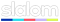# George Kosmidis

Microsoft MVP | Speaks of Azure & .NET | Founder of Munich .NET
Building tomorrow @# SQL Server: Weird behavior with CONTAINSTABLE, ISABOUT and weighted terms

by George Kosmidis / Published 11 years ago, modified 2 years ago

I came across the following weird behavior in an ISABOUT query in SQL SERVER, that involves the weight keyword and the final rank of the results. I want to describe this behavior here, just in case someone has a good explanation for it!

This posts assumes some basic knowledge of querying with full text search

The following bullets, are the sum up of the behavior. Notice how the results are reversed as the weight value goes down!

• weight(1): RANK of KEY 1 is 249
(results order 1,2,3)
• weight(0.8): RANK of KEY 1 is 321
(weight down => rank up, results order 1,2,3)
• weight(0.2): RANK of KEY 1 is 998
(weight down => rank up, results order 1,2,3)
• weight(0.17): RANK of KEY 1 is 802
(weight down => rank down, results order 2,3,1)
• weight(0.16): RANK of KEY 1 is 935
(weight down => rank up, results order 2,1,3)
• weight(0.01): RANK of KEY 1 is 50
(weight down => rank down, results order 3,2,1)

As you can see, from 0.2 to 0.17 ranking decreases and results are messed up! From 0.16 results are inverted (the weight values that reproduce this behaviour depend on terms, columns searched, etc).

Microsoft states here that the actual value of RANK is meaningless, but I am sure the results order isn’t!

Reproducing the behavior

These are the exact queries that I used to reproduce this behaviour:

QUERY 1 (weight 1): (Initial ranking)

``````SELECT * FROM
CONTAINSTABLE(FullTextTable, FullTextColumn, 'ISABOUT ("weird" weight (1) ) ')
ORDER BY RANK DESC, [KEY]``````

Results

``````   KEY   RANK
1    249
2    156
3    114
``````

QUERY 2 (weight 0.8): (Ranking increases, initial order is preserved)

``````SELECT * FROM
CONTAINSTABLE(FullTextTable, FullTextColumn, 'ISABOUT ("weird" weight (0.8) ) ')
ORDER BY RANK DESC, [KEY]``````

Results

``````   KEY    RANK
1     321
2     201
3     146
``````

QUERY 3 (weight 0.2): (Ranking increases, initial order is preserved)

``````SELECT * FROM
CONTAINSTABLE(FullTextTable, FullTextColumn, 'ISABOUT ("weird" weight (0.2) ) ')
ORDER BY RANK DESC, [KEY]``````

Results

``````   KEY   RANK
1    998
2    877
3    692
``````

QUERY 4 (weight 0.17): (Ranking decreases, best match is now last, inverted behavior for these terms begin at 0.17)

``````SELECT * FROM
CONTAINSTABLE(FullTextTable, FullTextColumn, 'ISABOUT ("weird" weight (0.17) ) ')
ORDER BY RANK DESC, [KEY]``````

Results

``````   KEY   RANK
2    960
3    958
1    802
``````

QUERY 5 (weight 0.16): (Ranking increases, best match is now second)

``````SELECT * FROM
CONTAINSTABLE(FullTextTable, FullTextColumn, 'ISABOUT ("weird" weight (0.16) ) ')
ORDER BY RANK DESC, [KEY]``````

Results

``````   KEY   RANK
2    978
1    935
3    841
``````

QUERY 6 (weight 0.01): (Ranking decreases, best match is last again)

``````SELECT * FROM
CONTAINSTABLE(FullTextTable, FullTextColumn, 'ISABOUT ("weird" weight (0.01) ) ')
ORDER BY RANK DESC, [KEY]``````

Results

``````   KEY   RANK
3    105
2     77
1     50
``````

This of course causes major problems when you use a custom “word-breaker”, creating something like this:

``````CONTAINSTABLE(FullTextTable, FullTextColumn,
"wordA wordB wordC" weight (0.8),
"wordA*" NEAR "wordB*" NEAR "wordC*" weight (0.6),
"wordA*" weight (0.1),
"wordB*" weight (0.1),
"wordC*" weight (0.1)
)'
)``````

But for now, and until a better solution is found, I changed the algorithm of the custom word-breaker to always use weights above 0.2!